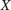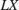# Solve Matrix Equation Using Forward Substitution

Solve a matrix equation using the Forward Substitution block.

Open and run the model. The model solves the equationusing the Forward Substitution block. The block acceptsandmatrices as inputs, and outputs the solution matrix.You can verify the solution by using the Matrix Multiply block to perform the multiplication, as shown in following model. The output matrixin this model equals the input matrixin the previous model.